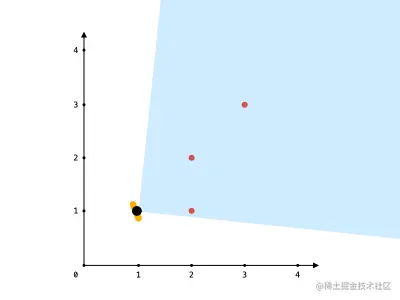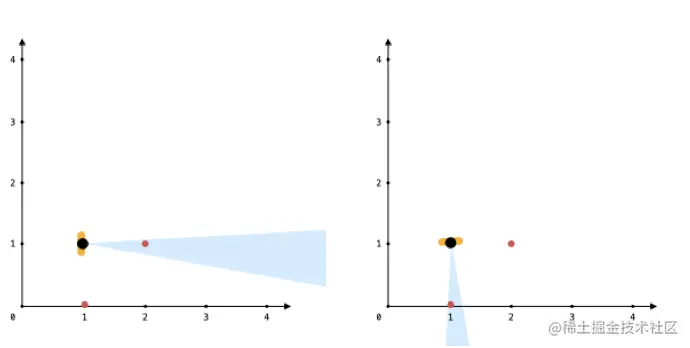# leetcode 1610. Maximum Number of Visible Points（python）

2022-02-01 06:23:56

「 This is my participation 11 The fourth of the yuegengwen challenge 23 God , Check out the activity details ：2021 One last more challenge

### describe

You are given an array points, an integer angle, and your location, where location = [posx, posy] and points[i] = [xi, yi] both denote integral coordinates on the X-Y plane.

Initially, you are facing directly east from your position. You cannot move from your position, but you can rotate. In other words, posx and posy cannot be changed. Your field of view in degrees is represented by angle, determining how wide you can see from any given view direction. Let d be the amount in degrees that you rotate counterclockwise. Then, your field of view is the inclusive range of angles [d - angle/2, d + angle/2].You can see some set of points if, for each point, the angle formed by the point, your position, and the immediate east direction from your position is in your field of view.

There can be multiple points at one coordinate. There may be points at your location, and you can always see these points regardless of your rotation. Points do not obstruct your vision to other points.

Return the maximum number of points you can see.

Example 1:``````Input: points = [[2,1],[2,2],[3,3]], angle = 90, location = [1,1]
Output: 3
Explanation: The shaded region represents your field of view. All points can be made visible in your field of view, including [3,3] even though [2,2] is in front and in the same line of sight.
Copy code ``````

Example 2:

``````Input: points = [[2,1],[2,2],[3,4],[1,1]], angle = 90, location = [1,1]
Output: 4
Explanation: All points can be made visible in your field of view, including the one at your location.
Copy code ``````

Example 3:``````Input: points = [[1,0],[2,1]], angle = 13, location = [1,1]
Output: 1
Explanation: You can only see one of the two points, as shown above.
Copy code ``````

Note:

• 1 <= points.length <= 10^5
• points[i].length == 2
• location.length == 2
• 0 <= angle < 360
• 0 <= posx, posy, xi, yi <= 100

### analysis

According to the meaning , Given a list points 、 An integer angle And your location , among location = [posx, posy] and points[i] = [xi, yi] All means X-Y Integral coordinates on the plane .

first , Stand in your position and face directly to the East . You can't move from your position , But you can rotate . let me put it another way ,posx and posy Can't change . The field of view in degrees consists of angle Express , Determines the width that can be seen from any given line of sight . Give Way d Indicates the number of degrees you rotate counterclockwise . then , Your vision is the angle [d - angle/2, d + angle/2] The scope of .

If for each point , From this point 、 Your position and the angle from your position directly to the East are in your view , You can see a collection of points . There can be multiple points on a coordinate . Your location may be a little , No matter how you rotate , You can always see these points . Points do not interfere with your view of other points . Return the maximum number of points you can see .

In fact, this question is relatively simple after reading , The general idea is to location As the origin , And then calculate points The angle between each point in the and the origin , It's all recorded angles in , Then use the sliding window idea to find angle The point with the most points in the range . There are two points in the title that need special attention ：

• The first is to calculate the included angle , The scope of attention is [0, 2*pi] , But maybe in 0 There may be an angle in line with the meaning of the topic up and down around the degree , So we need to angles Then expand each element and add 2*pi Value , Can be said [2*pi, 4*pi] The scope of the
• The second is the precision of decimals , Must be considered in place , After all, the computer is a floating point number when calculating the included angle , If the accuracy is not enough, there may be errors in finding points
• The third is that some points are at the origin , These points can always be seen , Just add it directly to the result

``````class Solution(object):
def visiblePoints(self, points, angle, location):
"""
:type points: List[List[int]]
:type angle: int
:type location: List[int]
:rtype: int
"""
angles = []
pi = 3.1415926
origin = 0
for x, y in points:
dx = x - location
dy = y - location
if dx == 0 and dy == 0:
origin += 1
continue
alpha = atan2(dy, dx) + pi
angles.append(alpha)
angles.sort()
N = len(angles)
for i in range(N):
angles.append(angles[i] + 2 * pi)

result = j = 0
for i in range(2*N):
while j < 2 * N and angles[j] - angles[i] <= angle * 1.0 * pi / 180 + 0.0000001:
j += 1
result = max(result, j - i)
return result + origin

Copy code ``````

### Running results

``````Runtime: 2204 ms, faster than 43.70% of Python online submissions for Maximum Number of Visible Points.
Memory Usage: 56.3 MB, less than 36.97% of Python online submissions for Maximum Number of Visible Points.
Copy code ``````